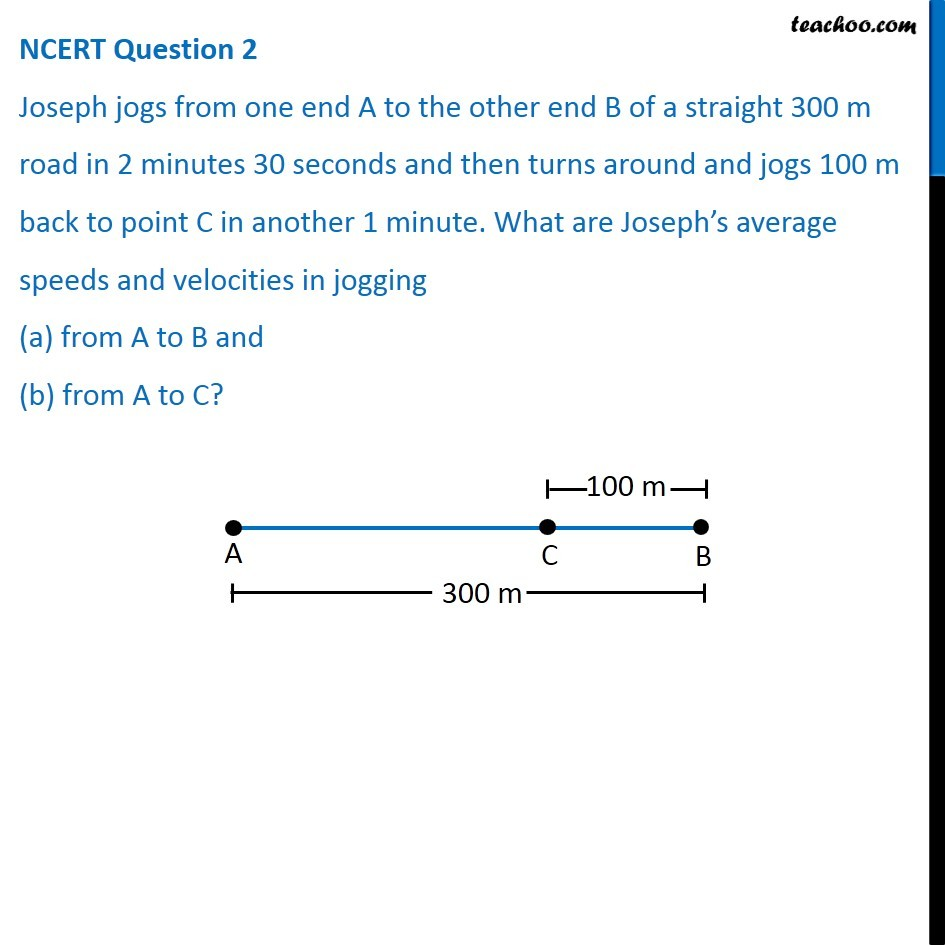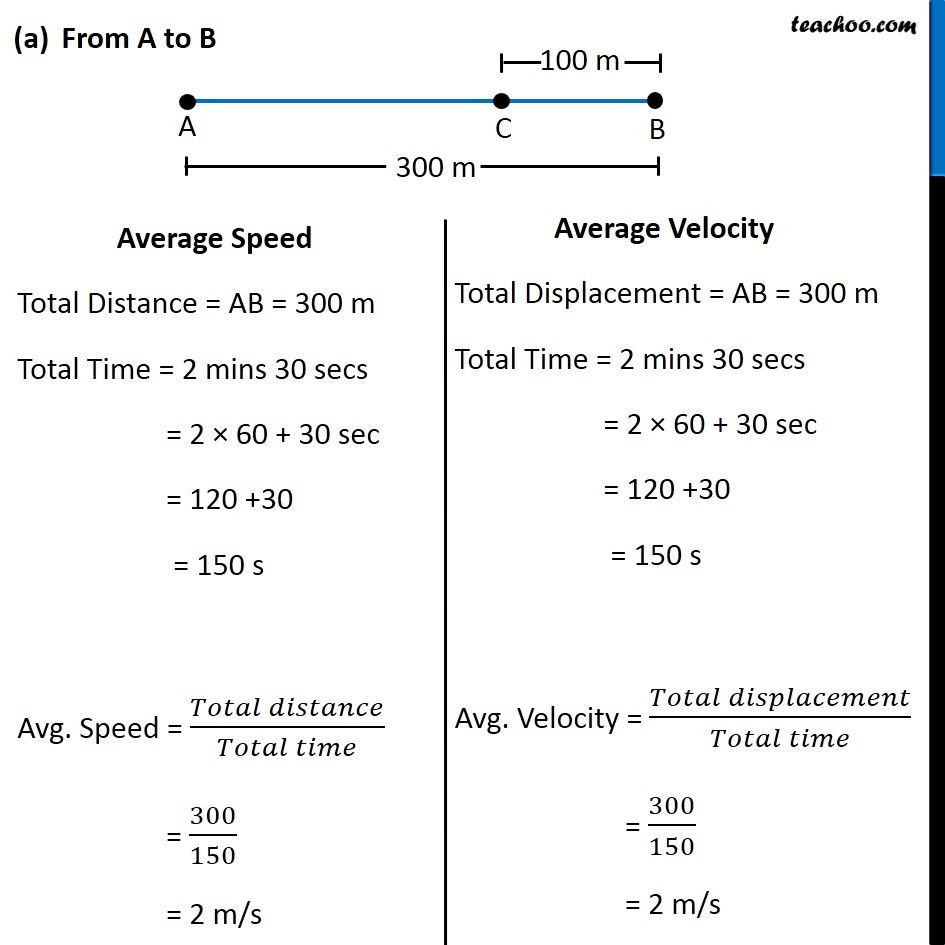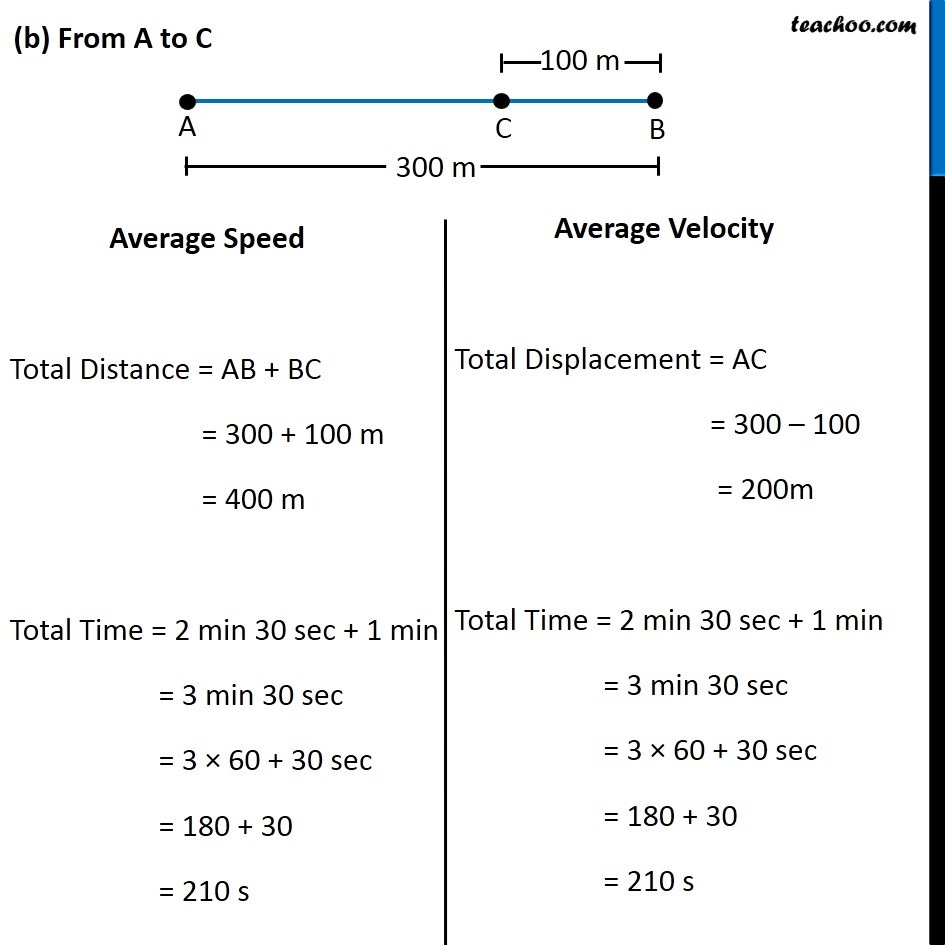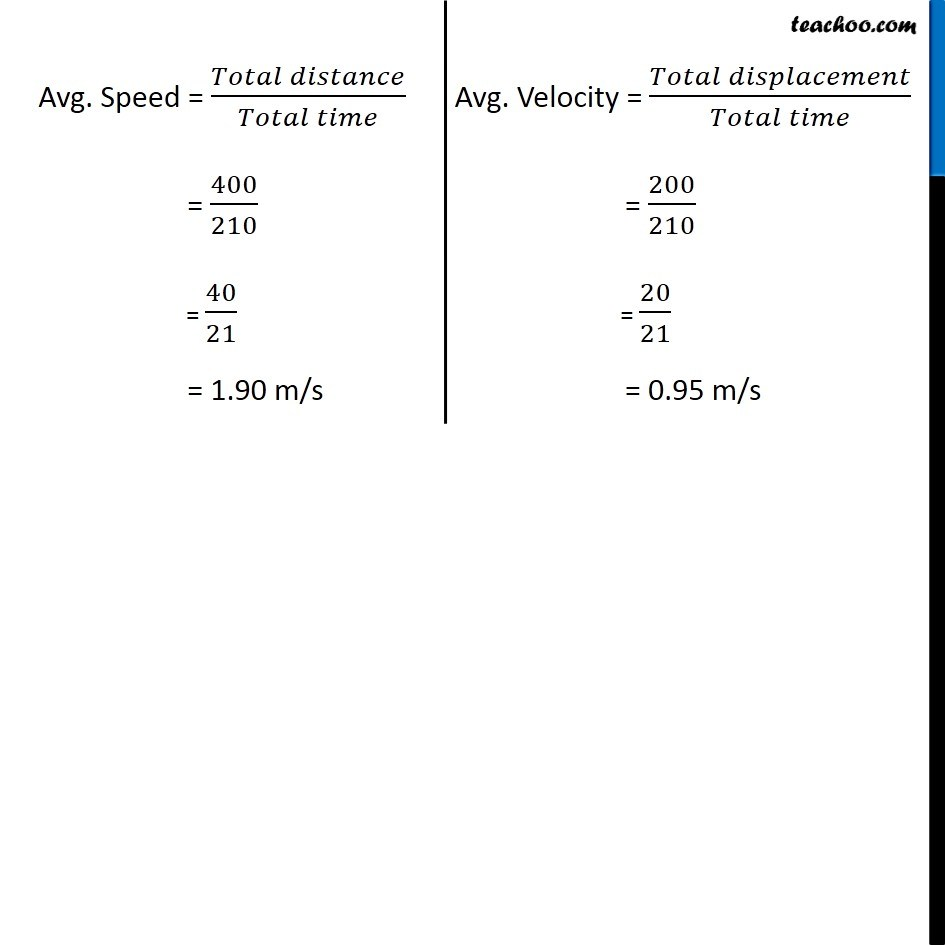NCERT Questions

Class 9
Chapter 8 Class 9 - MotionLearn in your speed, with individual attention - Teachoo Maths 1-on-1 Class

### Transcript

NCERT Question 2 Joseph jogs from one end A to the other end B of a straight 300 m road in 2 minutes 30 seconds and then turns around and jogs 100 m back to point C in another 1 minute. What are Joseph’s average speeds and velocities in jogging (a) from A to B and (b) from A to C? From A to B Average Speed Total Distance = AB = 300 m Total Time = 2 mins 30 secs = 2 × 60 + 30 sec = 120 +30 = 150 s Avg. Speed = (𝑇𝑜𝑡𝑎𝑙 𝑑𝑖𝑠𝑡𝑎𝑛𝑐𝑒)/(𝑇𝑜𝑡𝑎𝑙 𝑡𝑖𝑚𝑒) = 300/150 = 2 m/s Average Velocity Total Displacement = AB = 300 m Total Time = 2 mins 30 secs = 2 × 60 + 30 sec = 120 +30 = 150 s Avg. Velocity = (𝑇𝑜𝑡𝑎𝑙 𝑑𝑖𝑠𝑝𝑙𝑎𝑐𝑒𝑚𝑒𝑛𝑡)/(𝑇𝑜𝑡𝑎𝑙 𝑡𝑖𝑚𝑒) = 300/150 = 2 m/s (b) From A to C Average Speed Total Distance = AB + BC = 300 + 100 m = 400 m Total Time = 2 min 30 sec + 1 min = 3 min 30 sec = 3 × 60 + 30 sec = 180 + 30 = 210 s Average Velocity Total Displacement = AC = 300 – 100 = 200m Total Time = 2 min 30 sec + 1 min = 3 min 30 sec = 3 × 60 + 30 sec = 180 + 30 = 210 s Avg. Speed = (𝑇𝑜𝑡𝑎𝑙 𝑑𝑖𝑠𝑡𝑎𝑛𝑐𝑒)/(𝑇𝑜𝑡𝑎𝑙 𝑡𝑖𝑚𝑒) = 400/210 = 40/21 = 1.90 m/s Avg. Velocity = (𝑇𝑜𝑡𝑎𝑙 𝑑𝑖𝑠𝑝𝑙𝑎𝑐𝑒𝑚𝑒𝑛𝑡)/(𝑇𝑜𝑡𝑎𝑙 𝑡𝑖𝑚𝑒) = 200/210 = 20/21 = 0.95 m/s